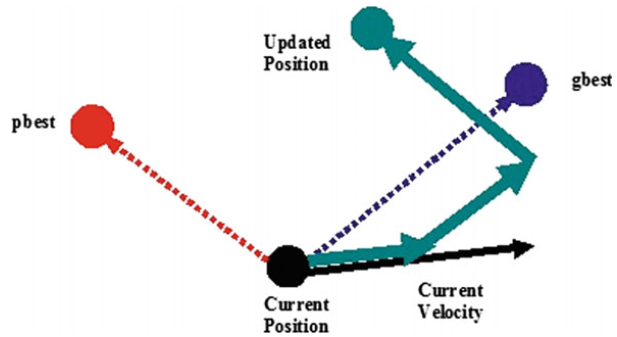# 【优化求解】PSO货物配装问题最优化【Matlab 348期】

## 一、简介

1.1 粒子群优化1.1.1 算法思想

1.1.2 粒子群优化过程

v i d t + 1 = v i d t + c 1 r 1 ( p i d t − x i d t ) + c 2 r 2 ( p g d t − x i d t ) (1) v_{id}^{t + 1} = v_{id}^t + {c_1}{r_1}\left( {p_{id}^t - x_{id}^t} \right) + {c_2}{r_2}\left( {p_{gd}^t - x_{id}^t} \right)\tag 1vidt+1​=vidt​+c1​r1​(pidt​−xidt​)+c2​r2​(pgdt​−xidt​)(1)

x i d t + 1 = x i d t + v i d t + 1 (2) x_{id}^{t + 1} = x_{id}^t + v_{id}^{t + 1}\tag 2xidt+1​=xidt​+vidt+1​(2)

1.1.3 解读更新等式1.2 粒子群优化中的参数

## 二、源代码

%clear all;
tic
clc;
%format long;
%------给定初始化条件----------------------------------------------
c1=2;             %学习因子1
c2=2;             %学习因子2
w=0.7;              %惯性权重
MaxDT=20;            %最大迭代次数
HX=10;
D=8;                  %搜索空间维数（未知数个数）
N=40;                  %初始化群体个体数目
%K=10^6;           %设置精度(在已知最小值时候用)
M=110;%货车载重
V=250;%货车容积
weight=[64,52,50,41,22,20,14,2];%物体重量
volume=[110,108,96,80,49,50,40,7];%物体体积
%load('psodata.mat');
%------初始化种群的个体(可以在这里限定位置和速度的范围)------------
x=round(rand([N D]));  %随机初始化位置
v=rand([N D]);  %随机初始化速度
%------先计算各个粒子的适应度，并初始化Pi和Pg----------------------
for i=1:N
plbest=fitness(x(i,:),D,M,V,weight,volume);%plbest表示个体最优值
pxbest=x(i,:);%pxbest表示个体最优位置
end
gxbest=x(1,:);             %gxbest为全局最优位置
for i=2:N
if fitness(x(i,:),D,M,V,weight,volume)>fitness(gxbest,D,M,V,weight,volume)
gxbest=x(i,:);
end
end
%fitness(gxbest,D,M,V,weight,volume)
%%------进入主要循环，按照公式依次迭代，直到满足精度要求------------
sumv=0;
summ=0;
for H=1:HX
Sum=0;
SUM=0;
for t=1:MaxDT
for i=1:N
v(i,:)=w*v(i,:)+c1*rand()*(pxbest-floor(x(i,:)))+c2*rand()*(gxbest-floor(x(i,:)));
x(i,:)=x(i,:)+v(i,:);
%%用于把每个分量取为0和1
for j=1:D
if rand()<=1/(1+exp(-v(i,j))) %Sigmoid函数
x(i,j)=0;
else
x(i,j)=1;
end
end
%x(i,:)
x1=crossover(x(i,:),gxbest,D);%把当前粒子与全局最优粒子的位置进行交叉
%x2=crossover(x1,y,D);
x3=mutation(x1);%把当前粒子的位置进行变异
if fitness(x3,D,M,V,weight,volume)>plbest
plbest=fitness(x3,D,M,V,weight,volume);
pxbest=x3;
end
end
if plbest>fitness(gxbest,D,M,V,weight,volume)
gxbest=pxbest;
end
glbest=fitness(gxbest,D,M,V,weight,volume);%glbest表示全局最优值
Sum=Sum+glbest(1);
aveg1=Sum/t;%体积平均
SUM=SUM+glbest(2);
aveg2=SUM/t;%载重平均
Z1(t)=aveg1;
Z2(t)=aveg2;
% X(t)=Pbest(1);
%Y(t)=Pbest(2);
%Q(t)=Pbest(3);
end
%%----------------最后给出计算结果-----------------------------
disp('*************************************************************')
disp('函数的全局最优位置为：')
Solution=gxbest
disp('最后得到的优化极值为：')
Result=fitness(gxbest,D,M,V,weight,volume)
WV=Result(1)
WM=Result(2)
Z1(H)=WV;
Z2(H)=WM;
%disp('箱子的体积利用率：')
sumv=sumv+WV;
%disp('货车的载重利用率：')
summ=summ+WM;
end
for i=1:N
plbest=fitness(x(i,:),D,M,V,weight,volume);%%plbest表示个体最优值
pxbest=x(i,:);%pxbest表示个体最优位置
end
gxbest=x(1,:);             %gxbest为全局最优位置
for i=2:N
if fitness(x(i,:),D,M,V,weight,volume)>fitness(gxbest,D,M,V,weight,volume)
gxbest=x(i,:);
end
end
%fitness(pg,D,M,V,weight,volume)
%%------进入主要循环，按照公式依次迭代，直到满足精度要求------------
Sum=0;
SUM=0;
for t=1:MaxDT
for i=1:N
v(i,:)=w*v(i,:)+c1*rand()*(pxbest-floor(x(i,:)))+c2*rand()*(gxbest-floor(x(i,:)));
x(i,:)=x(i,:)+v(i,:);
%用于把每个分量取为0和1
for j=1:D
if rand()<=1/(1+exp(-v(i,j))) %Sigmoid函数
x(i,j)=0;
else
x(i,j)=1;
end
end

if fitness(x(i,:),D,M,V,weight,volume)>plbest
plbest=fitness(x(i,:),D,M,V,weight,volume);

end
if plbest>fitness(gxbest,D,M,V,weight,volume)
gxbest=pxbest;
end
glbest=fitness(gxbest,D,M,V,weight,volume);%glbest表示全局最优值
Sum=Sum+glbest(1);
aveg1=Sum/t;%体积平均
SUM=SUM+glbest(2);
aveg2=SUM/t;%载重平均
Z3(t)=aveg1;
Z4(t)=aveg2;
%X(t)=Pbest(1);
%Y(t)=Pbest(2);
%Q(t)=Pbest(3);
end


## 三、运行结果## 四、备注

07-2212802-2788
03-04494
03-18189
03-0146
03-1848
03-18312
03-03324
03-04114
03-1840
02-27122
03-04103
03-18100
03-04495
03-04105
03-03125
03-1964
03-04128
03-0476
©️2020 CSDN 皮肤主题: 游动-白 设计师:白松林